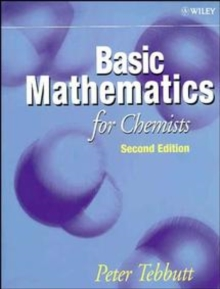Supporting your high street Find out how »
• My Account# Basic Mathematics for Chemists Paperback / softback

#### Description

Basic Mathematics for Chemists aims to teach the maths that chemists need to know through the use of applications, data, examples and problems all drawn from chemistry.

The author demystifies the maths, and shows how, where and why it is used in chemistry.

The text assumes little prior knowledge of maths and starts from basic mathematical principles, including understanding equations, notation, basic functions and their priorities.

It then covers more specialised functions such as logarithms and trigonometric functions before presenting chapters on calculus.

In this edition, there is a new chapter on vectors and matrices.

FEATURES Written by a chemist for chemists .Many examples, problems and applications. Gentle introduction to the maths chemists needs to know New chapter on vectors and matrices. Fully worked examples and problems provided within each chapter CONTENTS: Preface; Equations, Functions and Graphs; Special Functions; Practical Statistics; Differential Calculus; Integral Calculus; Differential Equations; Statistics for Theoretical Chemistry; Complex Numbers, Vectors, Determinants and Matrices; Appendix 1: The Greek Alphabet; Appendix 2: Numerical Answers to Problems.

#### Information

• Format: Paperback / softback
• Pages: 292 pages
• Publisher: John Wiley & Sons Inc
• Publication Date:
• Category: Maths for scientists
• ISBN: 9780471972846

£59.25

£50.55

on all orders

###### Pick up orders

from local bookshops